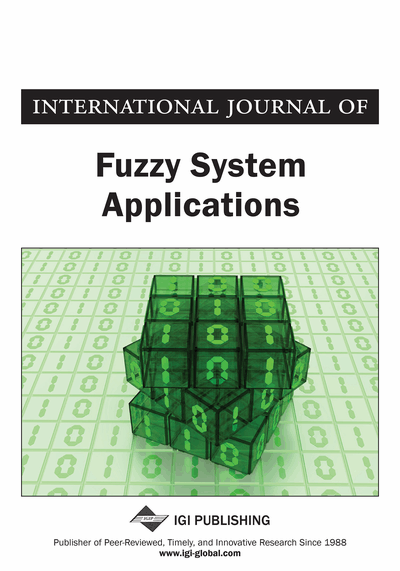# Contrasting Correlation Coefficient with Distance Measure in Interval Valued Intuitionistic Trapezoidal Fuzzy MAGDM Problems

John P. Robinson
DOI: 10.4018/IJFSA.2016100102
OnDemand:
(Individual Articles)
Available
\$37.50
No Current Special Offers

## Abstract

In this paper, Multiple Attribute Group Decision Making (MAGDM) problems in which the data is of the form of Interval Valued Intuitionistic Trapezoidal Fuzzy Numbers (IVITzFNs) is presented. Some operational laws of IVITzFNs are introduced. Then some new aggregation operators including interval valued Intuitionistic Trapezoidal Fuzzy Weighted Averaging (IVITzFWA) operator, interval valued Intuitionistic Trapezoidal Fuzzy Ordered Weighted Averaging (IVITzFOWA) operator and Interval Intuitionistic Trapezoidal Fuzzy Hybrid Averaging (IVITzFHA) operator, are proposed and some desirable properties of these operators are studied, such as Commutativity, Idempotency and Monotonicity. A new distance function and correlation coefficient are proposed for IVITzFNs which will be utilized for ranking the alternatives in MAGDM problems. Finally, numerical illustrations are given to verify the developed approach and to demonstrate its practicality and effectiveness.
Article Preview
Top

## Introduction

Multiple Attribute Group Decision Making (MAGDM) problems are wide spread in real life situations. A MAGDM problem is to find a desirable solution from a finite number of feasible alternatives assessed on multiple attributes, both quantitative and qualitative. To choose a desirable solution, the decision maker often provides his/her preferred information in the form of numerical values, such as exact values, interval number values and fuzzy numbers. However, under many conditions, numerical values are inadequate or insufficient to model real-life decision problems. Indeed, human judgments including preference information may be stated in intuitionistic fuzzy information. Hence, MAGDM problems under intuitionistic fuzzy environment are an interesting area of study for researchers recently. Processing of MAGDM problems with intuitionistic fuzzy information or vague fuzzy information, sometimes, leads to attribute values taking the form of intuitionistic or vague fuzzy number, respectively. The information about attribute weights may sometimes be known, partially known or be completely unknown. MAGDM problems are assumed to have a predetermined, limited number of decision alternatives. Solving a MAGDM problem involves sorting and ranking, and can be viewed as an alternative method for combining information in a problem’s decision matrix together with additional information from the decision maker to determine a final ranking or selection from the alternatives. Besides the information contained in the decision matrix, all but the simplest MAGDM techniques require additional information from the decision matrix to arrive at a final ranking / selection.

In many situations decision makers have imprecise/vague information about alternatives with respect to attributes. One of the methods which describe imprecise cases is the fuzzy set (FS) introduced by Zadeh, (1965). It is well known that the conventional decision making analysis using different techniques and tools has been found to be inadequate to handle uncertainty of fuzzy data. To overcome this problem, the concept of fuzzy approach has been used in the evaluation of decision making systems. In real life, a person may consider that an object belongs to a set, to a certain degree, and also it is possible that he is not sure about it. In other words, the person has hesitation about the membership degree. In classical fuzzy set theory there is no means to incorporate this hesitation regarding the degree of suitability to which each alternative satisfies the decision maker’s requirement. To include the unknown degree in the membership function of fuzzy sets, Atanassov, (1986, 1989, 1994) introduced the concept of intuitionistic fuzzy sets (IFSs), which is the generalization of the concept of fuzzy sets (Atanassov, 1989). Out of several higher-order fuzzy sets, IFSs, first introduced by Atanassov, (1986) has been found to be compatible to deal with vagueness. The concept of IFS can be viewed as an alternative approach to define a fuzzy set in cases where the available information is not sufficient for the definition of an imprecise concept by means of a conventional fuzzy set. In fuzzy sets only the degree of acceptance is considered, but IFS is characterized by a membership function and a non-membership function, so that the sum of both values is less than one. Presently IFSs are being studied and used in different fields of science. Later, Atanassov & Gargov, (1989) further introduced the Interval Intuitionistic Trapezoidal Fuzzy Set (IVIFS), which is a generalization of the IFS. The fundamental characteristic of the IVIFS is that the values of its membership function and non-membership function are intervals rather than exact numbers.

## Complete Article List

Search this Journal:
Reset
Volume 13: 1 Issue (2024)
Volume 12: 1 Issue (2023)
Volume 11: 4 Issues (2022)
Volume 10: 4 Issues (2021)
Volume 9: 4 Issues (2020)
Volume 8: 4 Issues (2019)
Volume 7: 4 Issues (2018)
Volume 6: 4 Issues (2017)
Volume 5: 4 Issues (2016)
Volume 4: 4 Issues (2015)
Volume 3: 4 Issues (2013)
Volume 2: 4 Issues (2012)
Volume 1: 4 Issues (2011)
View Complete Journal Contents Listing